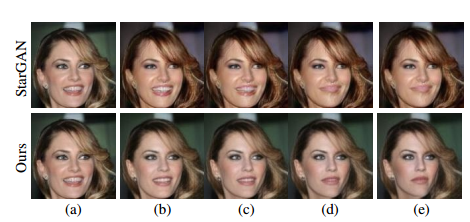• Latent Space Interpolation

## 解决的思路## 核心知识点

Decoder 和 Reconstruction 都是用 perceptual loss 训的。

$I_v(F_i, F_j) = F_i + \sum_{k=1}^c v^k T^k(F_j-F_i)$

// 我也不知道为啥公式要换一行才不会炸啊.. 我也很绝望啊